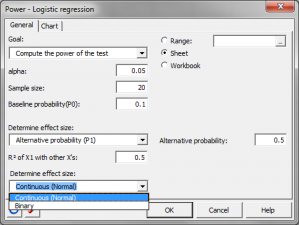# Statistical Power for logistic regression

Ensure optimal power or sample size using power analysis. Power for logistic regression is available in Excel using the XLSTAT statistical software.## Statistical Power for Logistic regression

XLSTAT-Base offers a tool to apply logistic regression. XLSTAT-Power estimates the power or calculates the necessary number of observations associated with this model. When testing a hypothesis using a statistical test, there are several decisions to take:

• The null hypothesis H0 and the alternative hypothesis Ha.
• The statistical test to use.
• The type I error also known as alpha. It occurs when one rejects the null hypothesis when it is true. It is set a priori for each test and is 5%.

The type II error or beta is less studied but is of great importance. In fact, it represents the probability that one does not reject the null hypothesis when it is false. We cannot fix it up front, but based on other parameters of the model we can try to minimize it. The power of a test is calculated as 1-beta and represents the probability that we reject the null hypothesis when it is false.

We therefore wish to maximize the power of the test. The XLSTAT-Power module calculates the power (and beta) when other parameters are known. For a given power, it also allows to calculate the sample size that is necessary to reach that power.

The statistical power calculations are usually done before the experiment is conducted. The main application of power calculations is to estimate the number of observations necessary to properly conduct an experiment. In the general framework of logistic regression model, the goal is to explain and predict the probability P that an event appends (usually Y=1). P is equal to: P = exp(β0 + β1X1 + … + βkXk) / [1 + exp(β0 + β1X1 + … + βkXk) ] We have: log(P/(1-P)) = β0 + β1X1 + … + βkXk The test used in XLSTAT-Power is based on the null hypothesis that the β1 coefficient is equal to 0. That means that the X1 explanatory variable has no effect on the model.

The hypothesis to be tested is:

• H0: β1 = 0
• Ha: β1 ≠ 0

## Calculation of the statistical power for logistic regression

Power is computed using an approximation which depends on the type of variable. If X1 is quantitative and has a normal distribution, the parameters of the approximation are:

• P0 (baseline probability): The probability that Y=1 when all explanatory variables are set to their mean value.
• P1 (alternative probability): The probability that X1 be equal to one standard error above its mean value, all other explanatory variables being at their mean value.
• Odds ratio: The ratio between the probability that Y=1, when X1 is equal to one standard deviation above its mean and the probability that Y=1 when X1 is at its mean value.
• The R² obtained with a regression between X1 and all the other explanatory variables included in the model.

If X1 is binary and follow a binomial distribution. Parameters of the approximation are:

• P0 (baseline probability): The probability that Y=1 when X1=0.
• P1 (alternative probability): The probability that Y=1 when X1 =1.
• Odds ratio: The ratio between the probability that Y=1, when X1 =1 and the probability that Y=1 when X1 =0.
• The R² obtained with a regression between X1 and all the other explanatory variables included in the model.
• The percentage of observations with X1 1.

These approximations depend on the normal distribution.

## Calculating sample size for logistic regression taking statistical power into account

To calculate the number of observations required, XLSTAT uses an algorithm that searches for the root of a function. It is called the Van Wijngaarden-Dekker-Brent algorithm (Brent, 1973). This algorithm is adapted to the case where the derivatives of the function are not known. It tries to find the root of:

power (N) - expected_power

We then obtain the size N such that the test has a power as close as possible to the desired power.### analyze your data with xlstat

14-day free trial

Included in

Related features